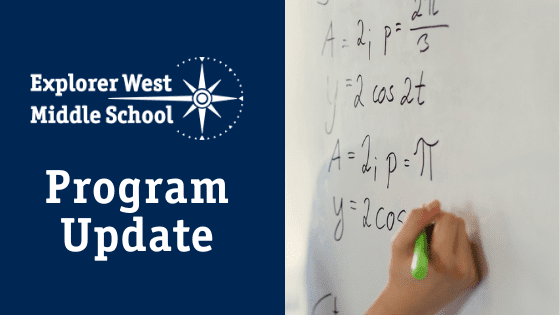# Math Program Updates at Explorer West6th Grade Math Update from Jon

The sixth graders have wrapped up a unit where they learned how to simplify algebraic expressions, and their new unit — solving equations — is now underway. The students have solved one-step equations and are beginning to work to solve two-step equations. From now until the end of the school year, they will continue working with more and more complex equations, and then they will pivot to a cumulative review of the topics they have learned this school year.

8th Grade Math Update from Jon

This school year, the eighth graders have learned how to graph linear equations, how to solve systems of linear equations, and how to solve quadratic equations, among other topics. They are reviewing everything they have learned in math class at Explorer West to prepare for a cumulative Final Exam. They will take a series of “practice tests” to figure out what they have mastered and what they need to study in the coming weeks.